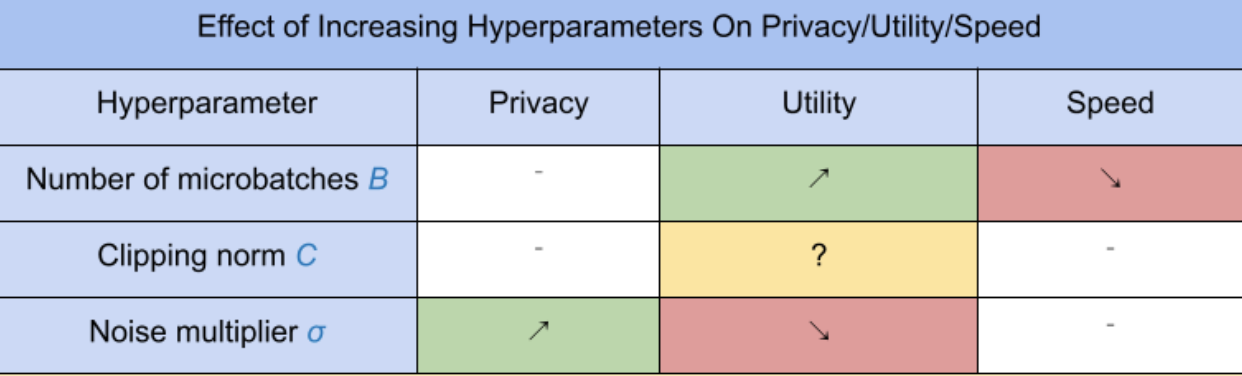Google I/O is a wrap! Catch up on TensorFlow sessions

# Get Started

This document assumes you are already familiar with differential privacy, and have determined that you would like to use TF Privacy to implement differential privacy guarantees in your model(s). If you’re not familiar with differential privacy, please review the overview page. After installing TF Privacy, get started by following these steps:

## 1. Choose a differentially private version of an existing Optimizer

If you’re currently using a TensorFlow optimizer, you will most likely want to select an Optimizer with the name DPKeras*Optimizer, such as [DPKerasAdamOptimizer] in [TF Privacy].

Optionally, you may try vectorized optimizers like [tf_privacy.VectorizedDPKerasAdamOptimizer]. for a possible speed improvement (in terms of global steps per second). The use of vectorized optimizers has been found to provide inconsistent speedups in experiments, but is not yet well understood. As before, you will most likely want to use an optimizer analogous to the one you're using now. These vectorized optimizers use Tensorflow's vectorized_map operator, which may not work with some other Tensorflow operators. If this is the case for you, please open an issue on the TF Privacy GitHub repository.

## 2. Compute loss for your input minibatch

When computing the loss for your input minibatch, make sure it is a vector with one entry per example, instead of aggregating it into a scalar. This is necessary since DP-SGD must be able to compute the loss for individual microbatches.

Train your model using the DP Optimizer (step 1) and vectorized loss (step 2). There are two options for doing this:

Once this is done, it’s recommended that you tune your hyperparameters. For a complete walkthrough see the classification privacy tutorial

## 4. Tune the DP-SGD hyperparameters

All tf_privacy optimizers take three additional hyperparameters:

• l2_norm_clip or $$C$$ - Clipping norm (the maximum Euclidean (L2) norm of each individual gradient computed per minibatch).
• noise_multiplier or $$σ$$ - Ratio of the standard deviation to the clipping norm.
• num_microbatches or $$B$$ - Number of microbatches into which each minibatch is split.

Generally, the lower the effective standard deviation $$σC / B$$, the better the performance of the trained model on its evaluation metrics.

The three new DP-SGD hyperparameters have the following effects and tradeoffs:

1. The number of microbatches $$B$$: Generally, increasing this will improve utility because it lowers the standard deviation of the noise. However, it will slow down training in terms of time.
2. The clipping norm $$C$$: Since the standard deviation of the noise scales with $$C$$, it is probably best to set $$C$$ to be some quantile (e.g. median, 75th percentile, 90th percentile) of the gradient norms. Having too large a value of $$C$$ adds unnecessarily large amounts of noise.
3. The noise multiplier $$σ$$: Of the three hyperparameters, the amount of privacy depends only on the noise multiplier. The larger the noise multiplier, the more privacy is obtained; however, this also comes with a loss of utility.

These tradeoffs between utility, privacy, and speed in terms of steps/second are summarized here:Follow these suggestions to find the optimal hyperparameters:

• Set $$C$$ to a quantile as recommended above. A value of 1.00 often works well.
• Set $$B$$ = 1, for maximum training speed.
• Experiment to find the largest value of σ that still gives acceptable utility. Generally, values of 0.01 or lower have been observed to work well.
• Once a suitable value of $$σ$$ is found, scale both $$B$$ and $$σ$$ by a constant to achieve a reasonable level of privacy.
[]
[]